###### eISSN: 2576-4500# Aeronautics and Aerospace Open Access JournalResearch Article Volume 2 Issue 1

# Finite element analysis of aircraft tire behaviour under overloaded aircraft landing phase

#### Ikpe Aniekan Essienubong, function clickButton(){ var name=document.getElementById('name').value; var descr=document.getElementById('descr').value; var unCopyslNo=document.getElementById('unCopyslNo').value; document.getElementById("mydiv").style.display = "none"; $.ajax({ type:"post", url:"https://medcraveonline.com/captchaCode/server_action", data: { 'name' :name, 'descr' :descr, 'unCopyslNo': unCopyslNo }, cache:false, success: function (html) { //alert('Data Send');$('#msg').html(html); } }); return false; } Verify Captcha Regret for the inconvenience: we are taking measures to prevent fraudulent form submissions by extractors and page crawlers. Please type the correct Captcha word to see email ID.function refreshPage(){ $("#mydiv").load(location.href + " #mydiv"); }$(document).ready(function () { //Disable cut copy paste $('#msg').bind('cut copy paste', function (e) { e.preventDefault(); }); //Disable mouse right click$("#msg").on("contextmenu",function(e){ return false; }); }); .noselect { -webkit-touch-callout: none; /* iOS Safari */ -webkit-user-select: none; /* Safari */ -khtml-user-select: none; /* Konqueror HTML */ -moz-user-select: none; /* Firefox */ -ms-user-select: none; /* Internet Explorer/Edge */ user-select: none; /* Non-prefixed version, currently supported by Chrome and Opera */ cursor: none; } Owunna Ikechukwu, Satope Paul

Department of Mechanical Engineering, Coventry University, UK

Correspondence: Ikpe Aniekan Essienubong, Masters in Coventry University, Mechanical Engineering, UK, Tel +2348125434634

Received: January 16, 2018 | Published: February 26, 2018

Citation: Essienubong IA, Ikechukwu O, Paul S, Finite element analysis of aircraft tire behaviour under overloaded aircraft landing phase. Aeron Aero Open Access J. 2018;2(1): 32-37. DOI: 10.15406/aaoaj.2018.02.00026

# Abstract

The effect of increasing aircraft landing weight on the tire during aircraft landing phase was investigated in this paper. 3D finite element tyre model was created and simulated using finite element tool (LS-DYNA). The landing scenario of 3.05m/s velocity as specified by European Aviation Safety Agency (EASA) standards was not specifically met but the modelling produce approximate landing scenario of 2.8m/s velocity. The landing velocity was one directional and it was modelled using the Boundary prescribed motion method in LS-DYNA. Applying 77300Kg (referenced landing weight of Airbus A321NEO) as initial load case, maximum von-mises stress of 270MPa was obtained for the modelled aircraft tire. Subsequent increase of the initial aircraft landing weight led to stress propagations across the tire model with increasing maximum von-mises stress value up to 20000MPa upon an applied force of 150000Kg exerted by the aircraft landing weight. It was observed that the tire had started undergoing deformation at aircraft landing weight above the initial weight of 114000Kg with maximum von-mises of 4360MPa at the point of landing, but this deformation which was characterize by cracks due to wear and tear effect of the tire thread had intensified by tearing the tire at aircraft landing weight of 126000Kg with maximum von-mises stress of 9620MPa. However at a landing weight of 150000Kg, von-stress distribution (20460MPa) across the tire structure was relatively high and this led to increase in the tearing/blowout effect on the tire. Hence, airlines should effect intensive checking routine in order to prevent the operation of overloaded aircraft, as this may not only damage the aircraft tire or its assembly but can result in unforeseen airplane crash.

Keywords: aircraft weigh, aircraft phase, tire, simulation, wear, stress deflection, traction wave

# Introduction

Theoretical evaluation

Tire rolling resistance coefficients (RRC) is the force resisting the motion when an aircraft tire rolls on asphalt surfaces during landing. This is given by the following equation 1 and 2;

$RR{C}_{1}=\frac{{F}_{x}}{{F}_{z}}$      (1)

$RR{C}_{2}=\frac{{M}_{y}}{r}}{{F}_{z}}$      (2)

Where, Fx is the longitudinal forces, Fz is the vertical loads, r is the rolling radius, My is the rolling resistance moment and RRC is the tire Rolling Resistance Coefficients.

Depending on the severity of landing impact and tire rolling resistance during an aircraft landing phase, the overloaded aircraft tire in attempt to overcome the forces exerted on it by the aircraft may be subjected to certain conditions such as logarithmic decrement (𝛿) given by equation 3;

$\delta =1n\left(\frac{{\theta }_{1}}{{\theta }_{2}}\right)$      (3)

Where the first two amplitudes (Ɵ1 and Ɵ2) after landing are recorded with respect to the times (t1 and t2) between these amplitudes.

Considering the time between amplitudes, the undamped (𝜔𝑛𝑟) and damped (𝜔𝑑𝑟) periods of vibration is given by equation 4 and 5 respectively;

${\omega }_{nr}=\frac{2\pi }{{\tau }_{d}\sqrt{1-{\xi }_{bz}{}^{2}}}$    (4)

${\omega }_{dr}=\frac{2\pi }{{\tau }_{d}}$      (5)

Wher e, ${\tau }_{d}$ is the damped period of vibration given by equation 6;

${\tau }_{d}={t}_{2}-{t}_{1}$         (6)

The over damped condition may be due to the upward and downward motion of the aircraft during landing. The energy will decay much like an under damped mechanical systems and the damping constant can be obtained by calculating the difference in oscillation amplitudes as time progresses. In this case, the damping ratio (𝜉) is given by equation 7,

$\xi =\frac{\delta }{\sqrt{4{\pi }^{2}-{\delta }^{2}}}$      (7)

The t1 and t2 represents the time of first and second peak for every damping period. Taking account of the undamped and damped periods of vibration, the critical damping constant (Cc) is given by equation 8;

${C}_{c}=2×{I}_{by}×{\omega }_{nr}$   (8)

Where Iby is the moment of inertia of the airplane tire belt.

Since the aircraft at the point of landing is subjected to periodic oscillation, the amplitude (A) which is the maximum displacement from equilibrium can be determine by rearranging the relationship expressed in equation 9;

$x=Α\mathrm{sin}\left(\omega t+\varphi \right)$      (9)

From equation 9, the amplitude (A) can be expressed as;

$Α=\frac{x}{\mathrm{sin}\left(\omega t+\varphi \right)}$        (10)

Where, x is the displacement (m), A is the amplitude (m),$Α=\frac{x}{\mathrm{sin}\left(\omega t+\varphi \right)}$ is the angular frequency (rad/s), t is time (s) and $\varphi$ is the phase shift (radians). The frequency (f) in terms of angular velocity is given by equation 11;

$f=\frac{\omega }{2\pi }$      (11)

Considering the oscillation amplitude and their effects, the aircraft tire may be prone to wear, crack propagation and critical tearing energy which is the energy released per unit area of crack surface growth as given by equation 12;

$T=-{\left(\frac{\partial W}{\partial A}\right)}_{\mathcal{l}}$       (12)

Where, T is the tearing energy (or energy release rate), W is the elastic energy stored in the aircraft tire, A is the area of one fracture surface of the crack and the suffix denotes$\mathcal{l}$ with constant displacement of the boundaries over which forces are applied. Hence, the interaction between the aircraft weight and the responding behaviour of the tire is given by the relationship in equation 13;

(13)

In this case, severe loading condition of the aircraft during landing may cause the aircraft tire to deflect, and this deflection depending on its severity may build up high stresses and cracks on the tire. However, this can subject the tire to intensive wear, distortion and tear effect if the overdamping frequency is continuous or if the vertically applied load in terms of the aircraft weight is excessive.14 The distortion can be determined using von-mises theory which helps in predicting yielding of a material when subjected to critical loading conditions. The yielding criteria for von-mises stress can be expressed by the yield stress relationship in equation 14;

(14)

Where ${\sigma }_{x},{\sigma }_{y},{\sigma }_{z}$ = Principal stresses in corresponding direction,${\tau }_{xy},{\tau }_{xz},{\tau }_{yz}$ ${\sigma }_{Y}$ = Shear stresses in corresponding planes and = yield stress.

# Materials and methods

Aircraft tire was modeled using LS-DYNA to address the effect of increasing aircraft landing weight on tire. Simulating the model shown in Figure 1, it was expected that the landing forces which is directly proportional to the critical stresses would cause further changes in the geometry of the tire. The tire computer model would make use of a computation method based on the finite element analysis technique and would use an explicit solver in order to extract the corresponding stresses resulting from the loading condition. The justification for adopting this computational method is due to the dynamics of interaction (between the aircraft weight, the tire and the asphalt surface) in the aircraft landing phase and stress wave propagation across the tire framework (Figure 1).

Furthermore, reference maximum landing weight (77,300kg) of Airbus A321NEO was used as the initial loading criteria,15 followed by subsequent loading values at increasing intervals. That is, when the loads (maximum aircraft landing weight) were applied, oscillation of the tire model occurred and each amplitude of oscillation was noted accordingly. However, three aircraft landing scenarios considered for this study included no contact with ground, point of landing and after landing but this study is focused on the point of landing (or point of contact with the ground) where the aircraft tire had full contact with the ground as presented in Figure 2.

Figure 2A represents a scenario where the aircraft has not yet landed. In addition, Figure 2B represents a scenario where the aircraft has just landed, while, Figure 2C represent an empty aircraft weight where the aircraft has landed with passengers and cargo evacuated.

Modelling software

Cambridge Engineering Software (CES), 2016 version was used to select the grade of rubber material for the tire model using. The modelling and simulation of the aircraft tyre were carried out using the explicit finite element software (LS-DYNA). Explicit finite element analysis can solve problems that require small time step in calculating the properties and the geometry of a body at a particular time during a simulation. This is possible because explicit analysis uses central difference method and return mapping algorithm for solving explicit problems and thus the expensive matrix inversions and numerical ite ration can be avoided in calculating the properties or geometry of a material at any particular time. LS-DYNA was chosen because it has the advantage of being a very good tool for explicit solver for dynamic transient analysis (those including blasting and high speed impact situations).16 The LS-DYNA PRIMER software and its associated packages were used in conjunction with the High Performance Computer (HPC). The analyses of the results generated were carried out using the LS-PREPOST software.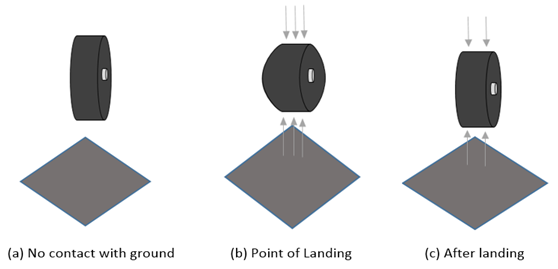Figure 2 Aircraft Landing Scenarios Considered for this Study.

# Results and discussion

Stress variation on tyre model with respect to increasing aircraft landing weight was simulated and the maximum and minimum von-mises stresses were recorded as shown in Table 1. Figure 3 is a graphical illustration of the von-mises stress values in response to the aircraft landing weight. Figures 4‒9 show the stress plot and their effects on the geometry of the aircraft tire at increasing aircraft weight.

As shown in Figure 10, the final pressure of the tyre was updated from 1.289 to 1.262 tonnes per cubic millimetre. The tyre is inflated gradually from 0 to 1.262 tonnes per cubic millimetre within 0.1second to help reduce the computational time of the whole model. The pressure is thereafter maintained from that point to ensure that the pressure of the tyre at inflation and after inflation remains constant. At constant pressure the tyre is expected to impact the rigid surface. The final pressure of the simulation of the model after all corrections have been made to the model obtained from previous studies gave a steady increase from 0 till it reaches 1.262 tonnes per cubic millimetre and then remained steady throughout the simulation. The various energy plots for the entire model is presented in Figure 11.

To determine if the model is stable, energy distribution plot for Kinetic Energy (KE), internal energy and the Total Energy (TE) was analysed for the tire model. For 0.1s, the kinetic energy was zero because the tyre was stationary for this period. Within the 0.1s the internal energy increases because of the inflation of the tyre. Since total energy is a summation of the kinetic energy and the internal energy, the total energy also increases with the increasing internal energy till it reaches 0.1s. At this point the tyre begins to move and the kinetic energy increases and the total energy changes with the changing kinetic energy since the internal energy remains somewhat stable for the remaining period of the simulation. The sudden increase in the velocity of the tyre causes a small vibration in the model. Figure 12 represents the maximum landing velocity obtained for the tire model.

The plot of velocity against time shows that the maximum velocity obtained from the simulation by Guo et al.7 was 1.5m/s this is way below the stipulated value expected for the impact of an aircraft tyre. After thorough remodelling of the velocity using *PRESCRIBED_BOUNDARY_MOTION the results obtained from the simulation gave a maximum landing velocity of 2.8m/s of 3.05m/s maximum landing velocity stipulated by EASA standards. With a maximum landing velocity of 2.8m/s, there is proximity with the EASA standard compared to 1.5m/s obtained by Guo et al.7Figure 3 Von-mises Stress Values in Response to the Aircraft Landing Weight.Figure 4 Stress Plot for Aircraft Tire under 77300Kg aircraft Landing Weight.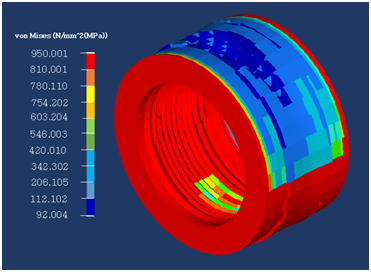Figure 5 Stress Plot for Aircraft Tire under 90200Kg aircraft Landing Weight.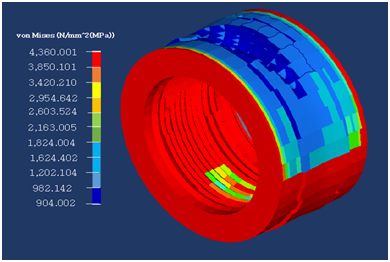Figure 6 Stress Plot for Aircraft Tire under 126000Kg aircraft Landing Weight.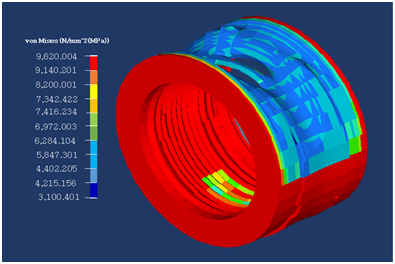Figure 7 Stress Plot for Aircraft Tire under 126000Kg aircraft Landing Weight.Figure 8 Stress Plot for Aircraft Tire under 134000Kg aircraft Landing Weight.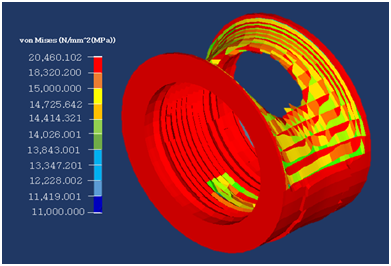Figure 9 Stress Plot for Aircraft Tire under 150000Kg aircraft Landing Weight.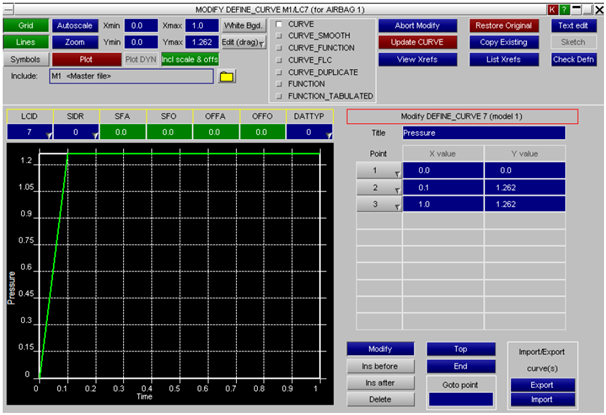Figure 10 Airbag Pressure model for aircraft tyre.Figure 12 Maximum Landing Velocity obtained for the Tire Model.
 Aircraft landing weight (Kg) Minimum von-mises stresses (Mpa) Maximum von-mises stresses (MPa) 77300 0 270 90200 92 950 114000 904 4360 126000 3100 9620 134000 7200 14730 150000 11000 20460

Table 1 Maximum and minimum von-mises stress for Tire Model

# Conclusion

By applying finite element modelling and analysis techniques, a research on the interaction of an aircraft tire with the ground during aircraft landing has been carried out. This study utilized *BOUNDARY_PRESCRIBED_MOTION methods to model the landing scenario of an aircraft at hard landing. Although the 2.8m/s maximum landing velocity for the simulated model was slightly lower than 3.05m/s EASA requirement, the 0.25m/s difference is optimum and can still meet the required performance. The 3-dimensional model developed and analysed have shown the effect of aircraft landing weight on the tire using finite element modelling. Maximum von-mises stress distribution across the tire model for simulated phases of the referenced aircraft landing weight fell between a minimum value of 270MPa and maximum value of 20460MPa with minimum aircraft landing weight of 77300Kg and maximum of 150000Kg respectively. This indicated that the higher the aircraft landing weight, the more damage the aircraft tire undergoes in terms of stress deformations and build up. Hence, airline operators should note that flying overloaded aircraft can have adverse negative effects on aircraft tire as well as aircraft landing phase.

# Acknowledgement

The authors of this manuscript would like to express their gratitude to Coventry University for providing the tools (used in achieving the objectives of this study) and guidance in terms of tutorial classes.

None.

# References©2018 Essienubong, et al. This is an open access article distributed under the terms of the, which permits unrestricted use, distribution, and build upon your work non-commercially.